# A Bayesian Adjustment to Rethink Priorities' Welfare Range Estimates

I was meditating on Rethink Priorities’ Welfare Range Estimates: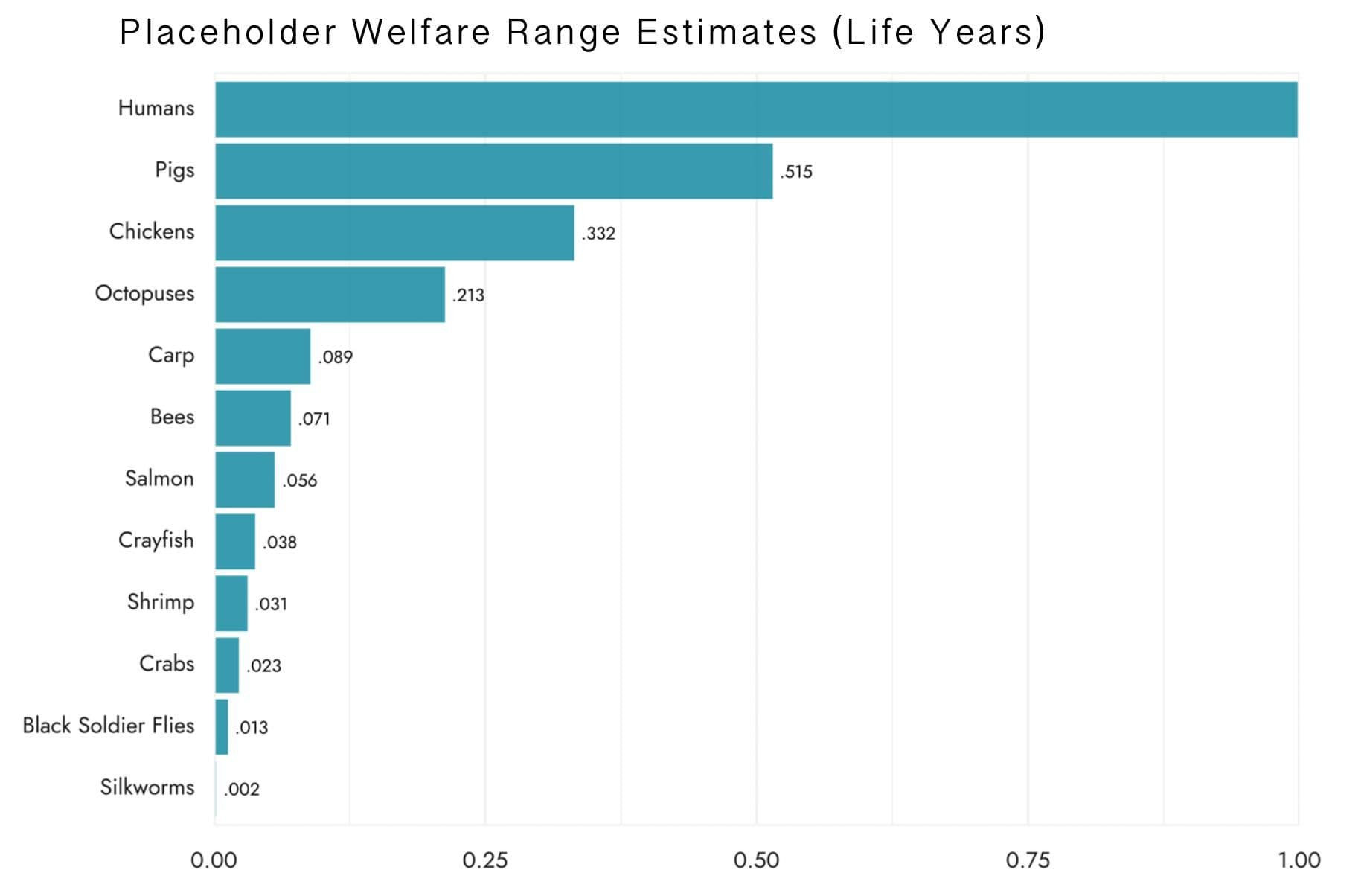Something didn’t feel right. Suddenly, an apparition of E. T. Jaynes manifested itself, and exclaimed: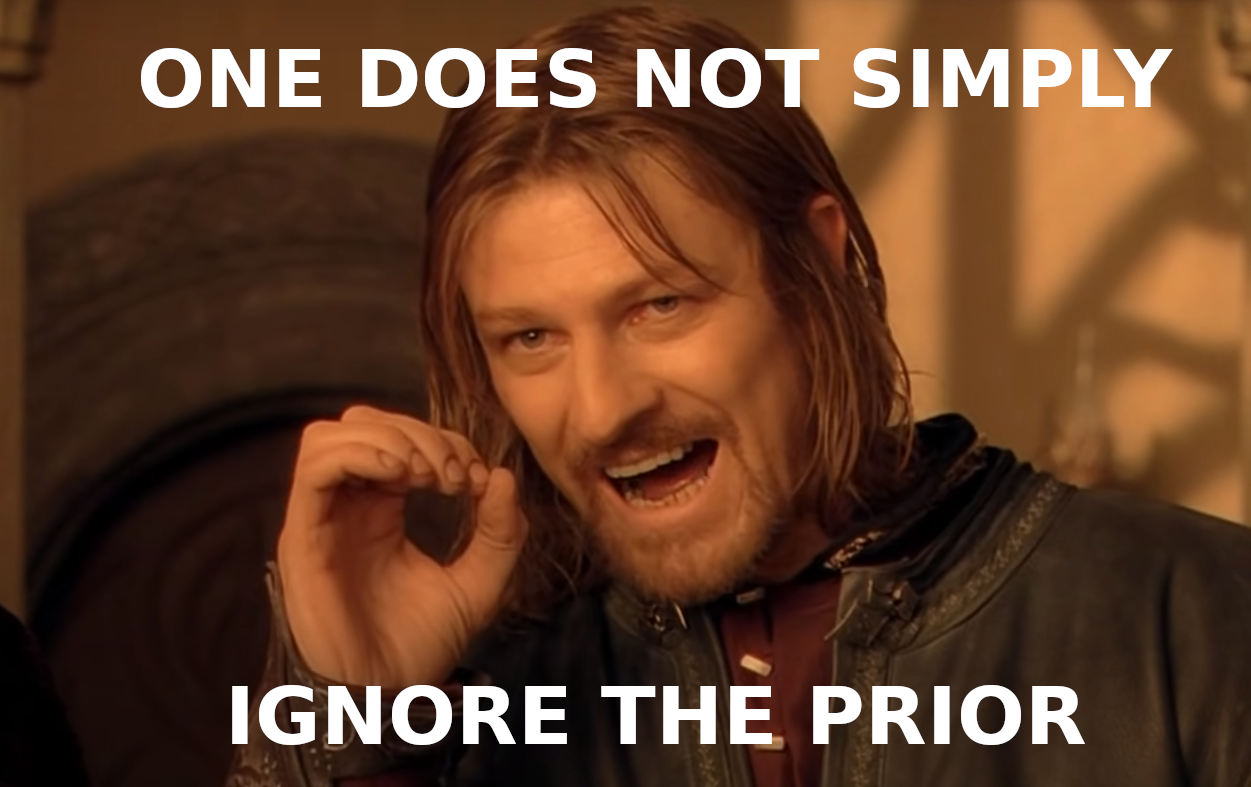The way was clear. I should:

1. Come up with a prior over welfare estimates
2. Come up with an estimate of how likely Rethink Priority’s estimates are at each point in the prior
3. Make a Bayesian update

### Three shortcuts on account of my laziness

To lighten my load, I took certain methodological shortcuts. First, I decided to use probabilities, rather than probability densities.

Taking probabilities instead of limits means that there is some additional clarity. Like, I can deduce Bayes Theorem from:

$$P(A \& B) = P(A) \cdot P(\text{B given A})$$ $$P(A \& B) = P(B) \cdot P(\text{A given B})$$

and therefore

$$P(A) \cdot P(\text{B given A}) = P(B) \cdot P(\text{A given B})$$

$$P(\text{B given A}) = P(B) \cdot \frac{P(\text{A given B})}{P(A) }$$

But for probability densities, is it the case that

$$d(A \& B) = d(A) \cdot d(\text{B given A})\text{?}$$

Well, yes, but I have to think about limits, wherein, as everyone knows, lie the workings of the devil. So I decided to use a probability distribution with 50k possible welfare points, rather than a probability density distribution.

The second shortcut I am taking is to interpret Rethink Priorities’s estimates as estimates of the relative value of humans and each species of animal—that is, to take their estimates as saying “a human is X times more valuable than a pig/chicken/shrimp/etc”. But RP explicitly notes that they are not that, they are just estimates of the range that welfare can take, from the worst experience to the best experience. You’d still have to adjust according to what proportion of that range is experienced, e.g., according to how much suffering a chicken in a factory farm experiences as a proportion of its maximum suffering.

And thirdly, I decided to do the Bayesian estimate based on RP’s point estimates, rather than on their 90% confidence intervals. I do feel bad about this, because I’ve been pushing for more distributions, so it feels a shame to ignore RP’s confidence intervals, which could be fitted into e.g., a beta or a lognormal distribution.

So in short, my three shortcuts are:

1. use probabilities instead of probability densities
2. wrongly interpret RP’s estimate as giving the tradeoff value between human and animals, rather than their welfare ranges.
3. use RP’s point estimates rather than fitting a distribution to their 90% confidence intervals.

These shortcuts mean that you can’t literally take this Bayesian estimate seriously. Rather, it’s an illustration of how a Bayesian adjustmetn would work. If you are making an important decision which depends on these estimates—e.g., if you are Open Philanthropy or Animal Charity Evaluators and trying to estimate the value of charities corresponding to the amount of animal suffering they prevent—then you should probably commission a complete version of this analysis.

### Focusing on chickens

For the rest of the post, I will focus on chickens. Updates for other animals should be similar, and focusing on one example makes my job easier.

Coming back to Rethink Priorities' estimates: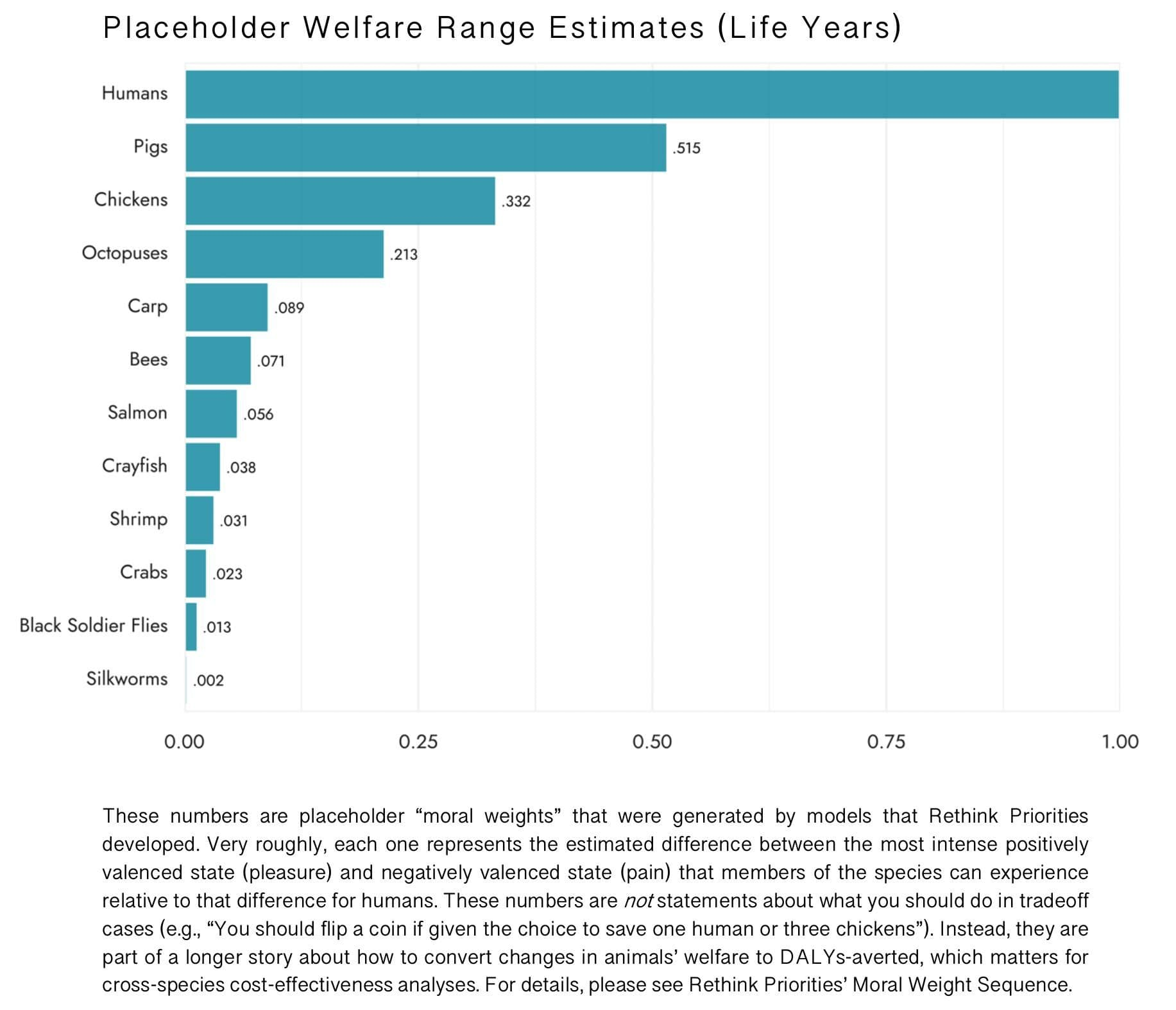Chickens have a welfare range 0.332, i.e., 33.2% as wide as that of humans, according to RP’s estimate.

Remember that RP has wide confidence intervals here, so that number in isolation produces a somewhat misleading impression: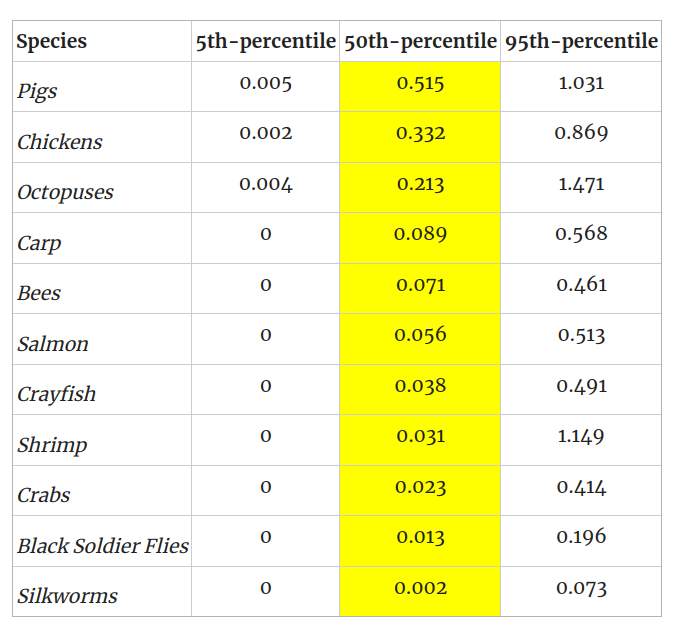### Constructing a prior

So, when I think about how I disvalue chickens' suffering in comparison to how I value human flourishing, moment to moment, I come to something like the following:

• a 40% that I don’t care about chicken suffering at all
• a 60% that I care about chicken suffering some relatively small amount, e.g., that I care about a chicken suffering $$\frac{1}{5000} \text{ to } \frac{1}{100}$$th as much as I care about a human being content, moment for moment

To those specifications, my prior thus looks like this: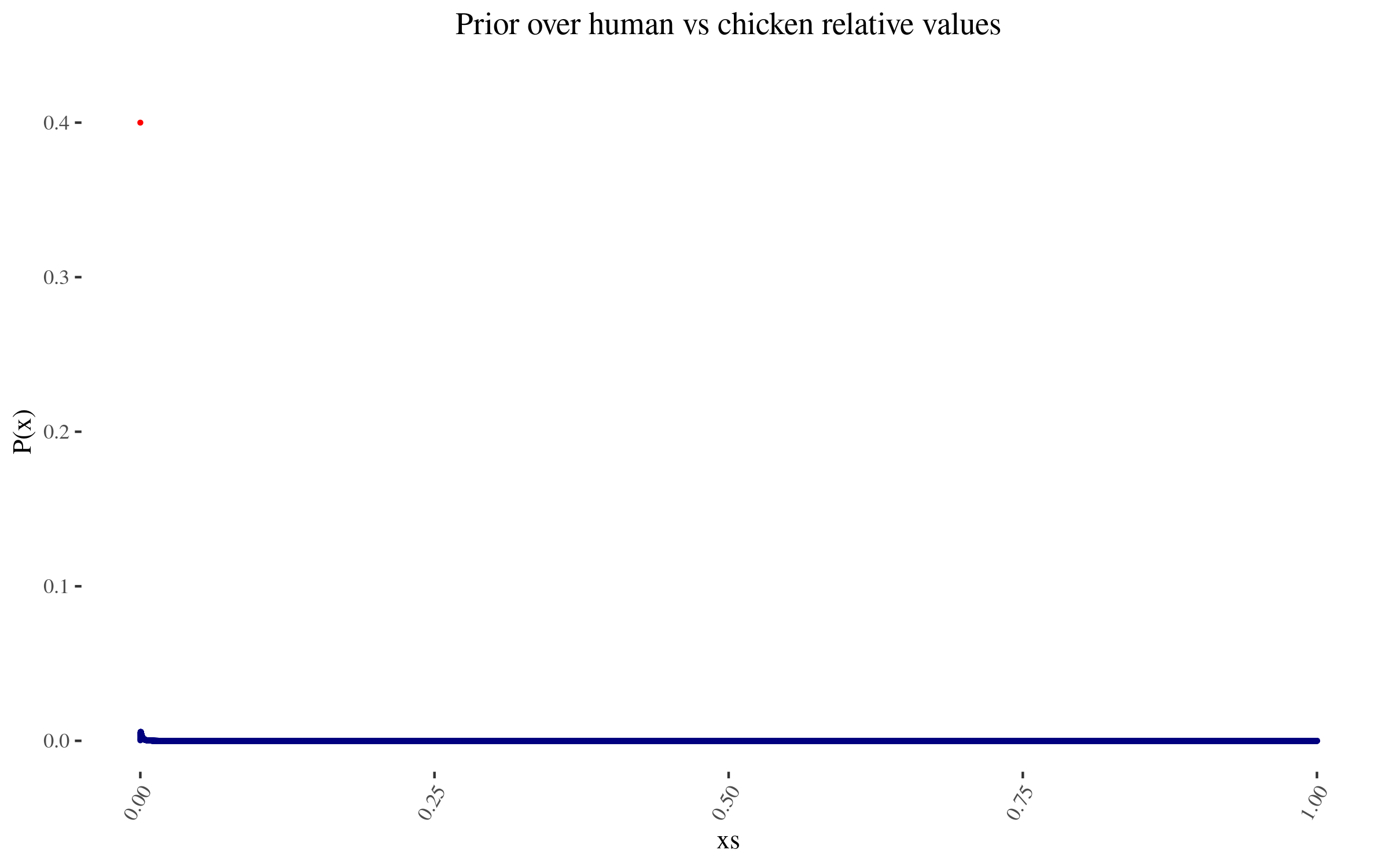The lone red point is the probability I assign to 0 value

Zooming in into the blue points, they look like this: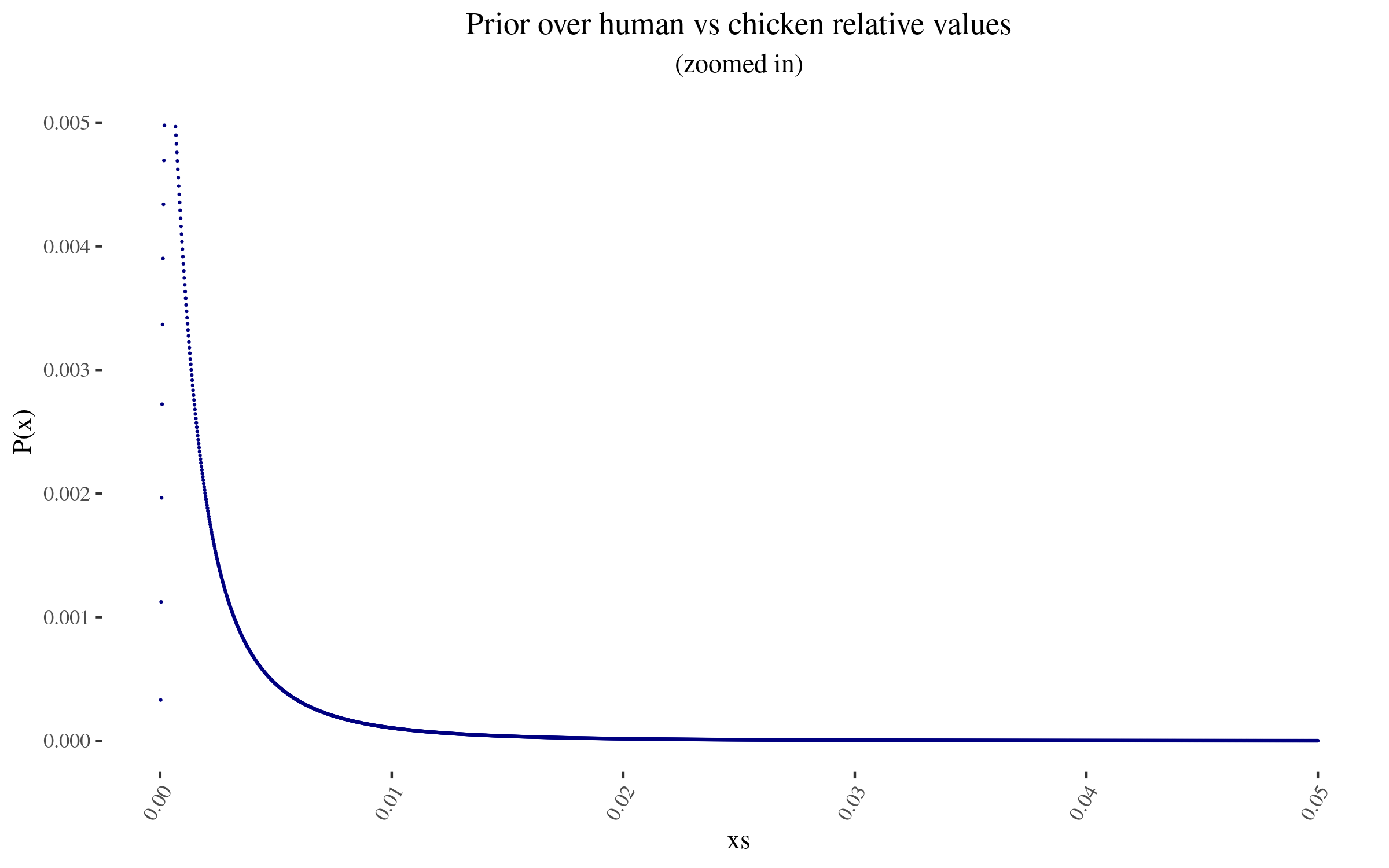As I mention before, note that I am not using a probability density, but rather lots of points. In particular, for this simulation, I’m using 50,000 points. This will become relevant later.

Code to produce this chart and subsequent updates can be seen here and here.

### Constructing the Bayes factor

Now, by $$x$$ let me represent the point “chickens are worth $$x$$ as much as human,”, and by $$h$$ let me represent “Rethink Priorities' investigation estimates that chickens are worth $$h$$ times as much as humans”

Per Bayes' theorem:

$$P(\text{x given h}) = P(x) \cdot \frac{P(\text{h given x})}{P(h) }$$

As a brief note, I’ve been writting “$$P( \text{A given B})$$” to make this more accessible to some readers. But in what follows I’ll use the normal notation “$$P(A|B)$$”, to mean the same thing, i.e., the probability of A given that we know that B is the case.

So now, I want to estimate $$P(\text{h given x})$$, or $$P(\text{h | x})$$. I will divide this estimate in two parts, conditioning on RP’s research having gone wrong ($$W$$), and on it having not gone wrong ($$\overline{W}$$):

$$P(h|x) = P(h | xW) \cdot P(W) + P(h | x \overline{W}) \cdot P(\overline{W})$$ $$P(h|x) = P(h | xW) \cdot P(W) + P(h | x \overline{W}) \cdot (1-P(W))$$

What’s the prior probability that RP’s research has gone wrong? I don’t know, it’s a tricky domain with a fair number of moving pieces. On the other hand, RP is generally competent. I’m going to say 50%. Note that this is the prior probability, before seing the output of the research. Ideally I’d have prerecorded this.

What’s $$P(h | xW)$$, the probability of getting $$h$$ if RP’s research has gone wrong? Well, I’m using 50,000 possible welfare values, so $$\frac{1}{50000}$$ might be a good enough approximation.

Conversely, what is, $$P(h | x\overline{W} )$$ the probability of getting $$h$$ if RP’s research has gone right? Well, this depends on how far away $$h$$ is from $$x$$.

• I’m going to say that if RP’s research had gone right, then $$h$$ should be within one order of magnitude to either side of $$x$$. If there are $$n(x)$$ welfare values within one order of magnitude of $$x$$, and $$h$$ is amongst them, then $$P(h|x\overline{W}) = \frac{1}{n(x)}$$
• Otherwise, if $$h$$ is far away from $$x$$, and we are conditioning both on $$x$$ being the correct moral value and on RP’s research being correct, then $$P(h | x\overline{W}) \approx 0$$.

With this, we can construct $$P(h | x)$$. It looks as follows: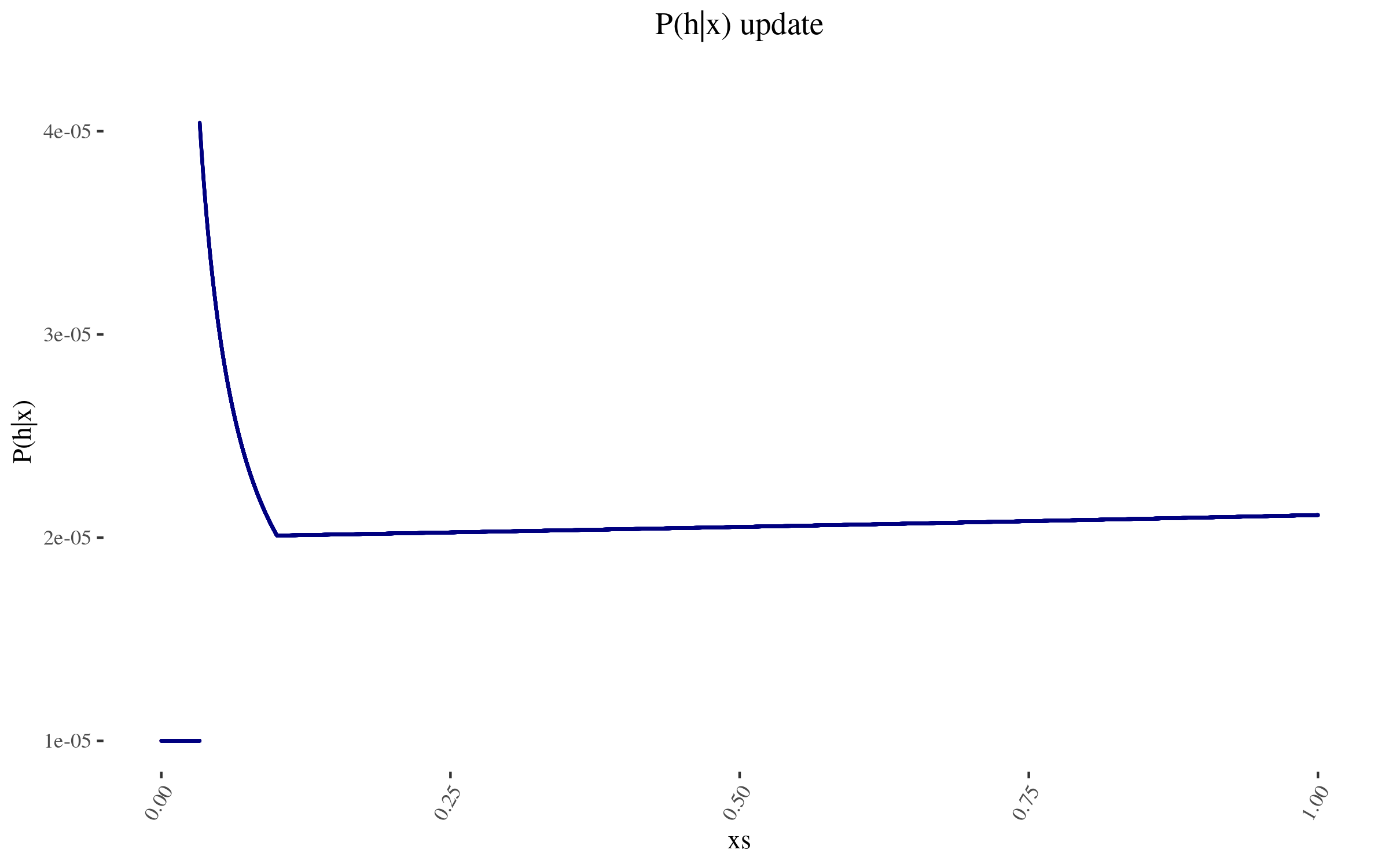The wonky increase at the end is because those points don’t have as many other points within one order of magnitude of their position. And the sudden drop off is at 0.0332, which is more than one order of magnitude away from the 0.332 estimate. To avoid this, I could have used a function smoother than “one order of magnitude away”, and I could have used a finer grained mesh, not just 50k points evenly distributed. But I don’t think this ends up mattering much.

Now, to complete the death star Bayesian update, we just need $$P(h)$$. We can easily get it through

$$P(h) = \sum P(h | x) \cdot P(x) = 1.005309 \cdot 10^{-5}$$

That is, our original probability that RP would end up with an estimate of 0.332, as opposed to any of the other 50,000 values we are modelling, was $$\approx 1.005309\cdot 10^{-5}$$. This would be a neat point to sanity check.

### Applying the Bayesian update

With $$P(x)$$ and $$\frac{P(h|x)}{P(h)}$$ in hand, we can now construct $$P(x|h)$$, and it looks as follows: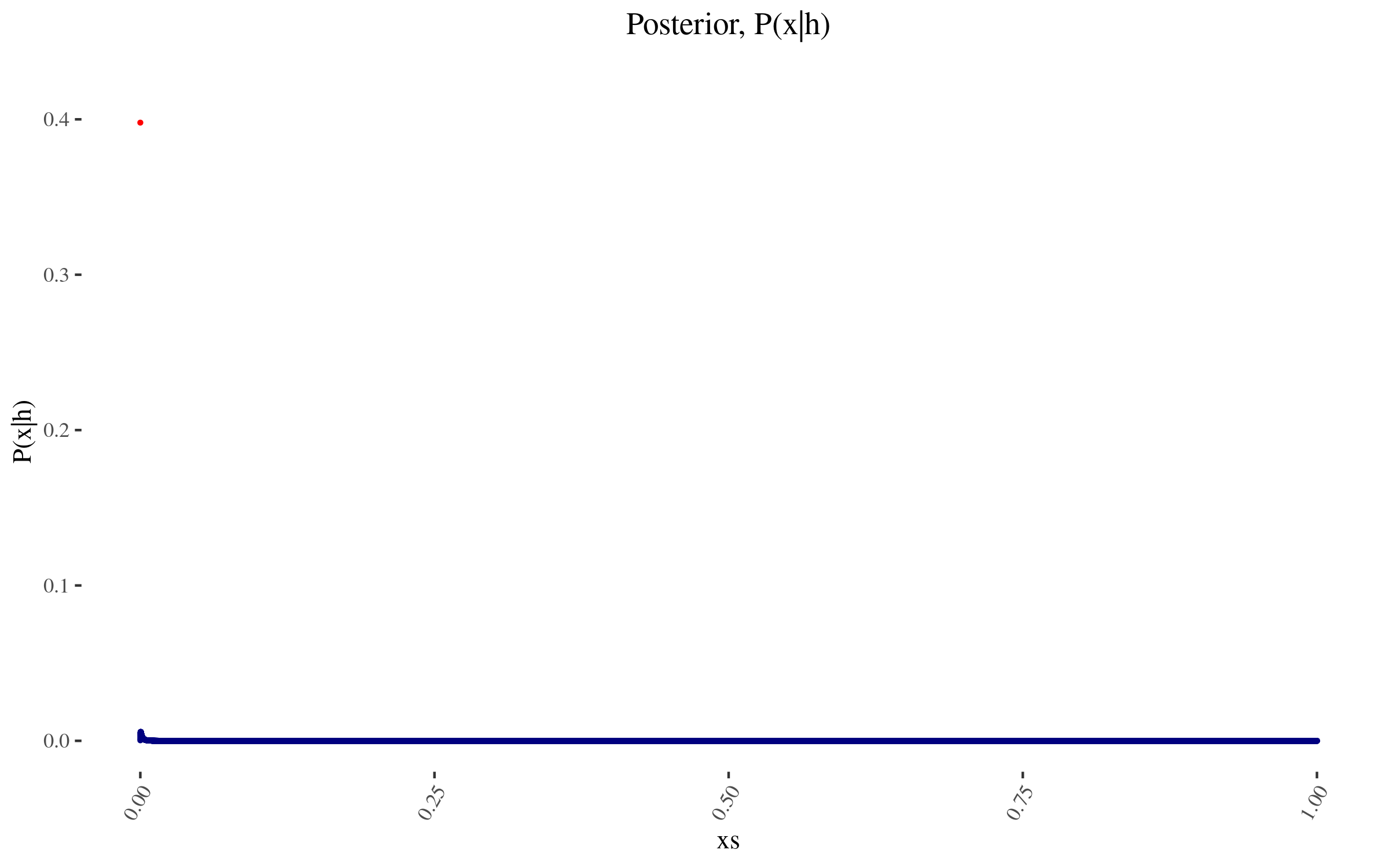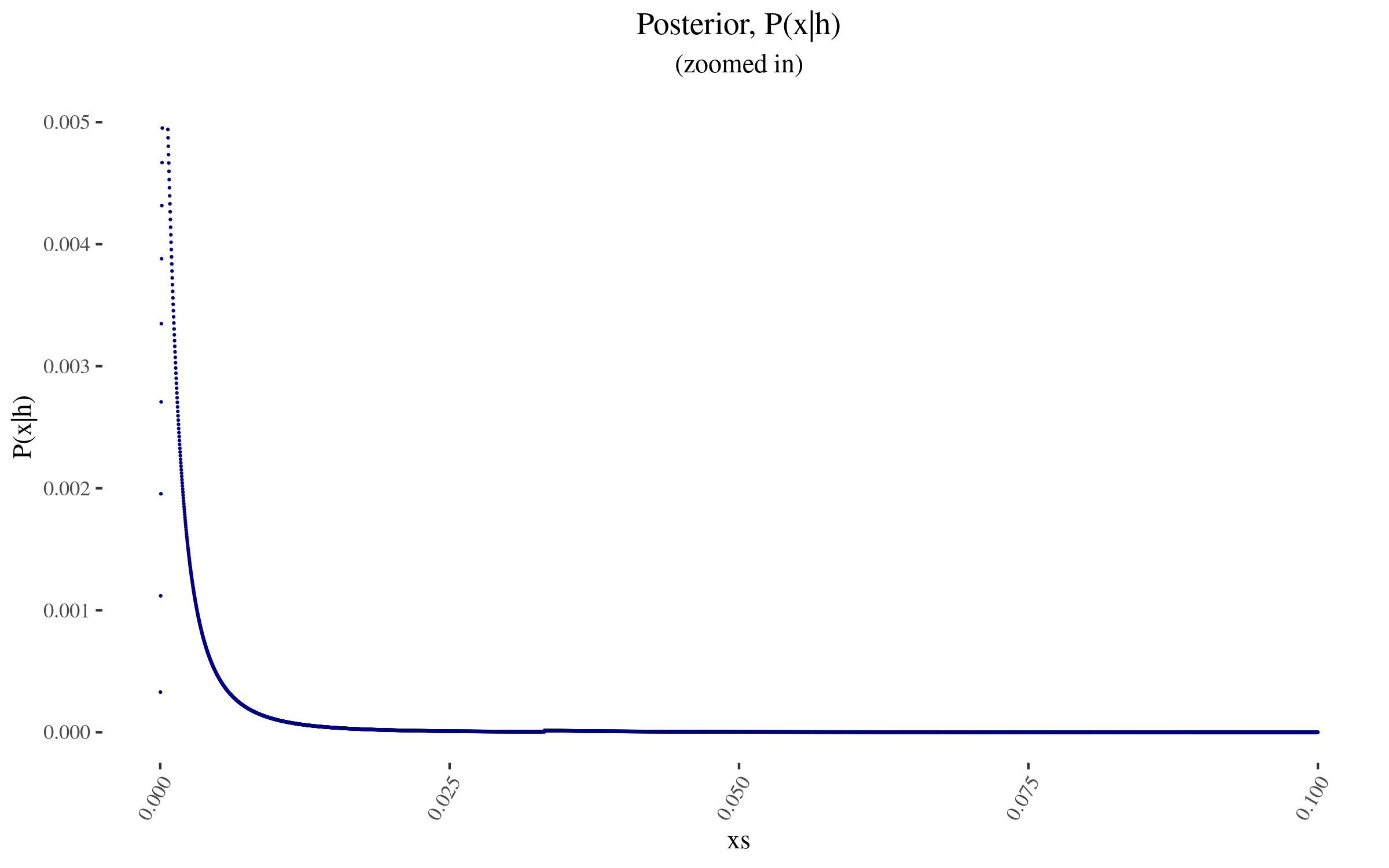### Getting a few indicators.

So for example, the probability assigned to 0 value moves from 40% to 39.788…%.

We can also calculate our prior and posterior average relative values, as

$$\text{Prior expected relative value} = \sum x \cdot P(x) \approx 0.00172$$ $$\text{Posterior expected relative value} = \sum x \cdot P(x | h) \approx 0.00196$$

We can also calculate the posterior probability that RP’s analysis is wrong. But, wrong in what sense? Well, wrong in the sense of incorrect at describing my, Nuño’s, values. But it may well be correct in terms of the tradition and philosophical assumptions that RP is working with, e.g,. some radical anti-speciesist hedonism that I don’t share.

So anyways, we can calculate

$$P(W | h) = \sum P(x) \cdot P(W | hx)$$

And we can calculate $$P(W|hx)$$ from Bayes:

$$P(W|hx) = P(W) \cdot \frac{P(h|Wx)}{P(h)}$$

But we have all the factors: $$P(W) = 0.5$$, $$P(h | Wx) = \frac{1}{50000}$$ and $$P(h) = 1.005309 \cdot 10^{-5}$$. Note that these factors are constant, so we don’t have to actually calculate the sum.

Anyways, in any case, it turns out that

$$P(W|h) = 0.5 \cdot \frac{\frac{1}{50000}}{1.005309 \cdot 10^{-5}} = 0.9947.. \approx 99.5\%$$

Note that I am in fact abusing RP’s estimates, because they are welfare ranges, not relative values. So it should pop out that they are wrong, because I didn’t go to the trouble of interpreting them correctly.

In any case, according to the initial prior I constructed, I end up fairly confident that RP’s estimate doesn’t correctly capture my values. One downside here is that I constructed a fairly confident prior. Am I really 97.5% confident (the left tail of a 95% confidence interval) that the moment-to-moment value of a chicken is below 1% of the moment-to-moment value of a human, according to my values? Well, yes. But if I wasn’t, maybe I could mix my initial lognormal prior with a uniform distribution.

### Conclusion

So, in conclusion, I presented a Bayesian adjustment to RP’s estimates of the welfare of different animals. The three moving pieces are:

• My prior
• My likelihood that RP is making a mistake in its analysis
• RP’s estimate

In its writeup, RP writes:

“I don’t share this project’s assumptions. Can’t I just ignore the results?”

We don’t think so. First, if unitarianism is false, then it would be reasonable to discount our estimates by some factor or other. However, the alternative—hierarchicalism, according to which some kinds of welfare matter more than others or some individuals’ welfare matters more than others’ welfare—is very hard to defend. (To see this, consider the many reviews of the most systematic defense of hierarchicalism, which identify deep problems with the proposal.)

Second, and as we’ve argued, rejecting hedonism might lead you to reduce our non-human animal estimates by ~⅔, but not by much more than that. This is because positively and negatively valenced experiences are very important even on most non-hedonist theories of welfare.

Relatedly, even if you reject both unitarianism and hedonism, our estimates would still serve as a baseline. A version of the Moral Weight Project with different philosophical assumptions would build on the methodology developed and implemented here—not start from scratch.

So my main takeaway is that that section is mostly wrong, you can totally ignore these results if you either:

• have a strong prior that you don’t care about certain animals, or, more specifically, that their welfare range is narrow.
• assign a high enough probability that RP’s analysis has gone wrong at some point

As for future steps, I imagine that at some point in its work, RP will post numerical estimates of human vs animal values, of which their welfare ranges are but one of many components. If so, I’ll probably redo this analysis with those factors. Besides that, it would also be neat to fit RP’s 90% confidence interval to a distribution, and update on that distribution, not only on their point estimate.

But to leave on a positive note, I see making a Bayesian adjustment like the above as a necessary final step, but one that is very generic and that doesn’t require the deep expertise and time-intensive effort that RP has been putting into its welfare estimates. So RP has still been producing some very informative estimates, that I hope will influence decisions on this topic.

### Acknowledgements

Thanks to Rethink Priorities for publishing their estimates so that I and others can play around with them. Generally, I’m able to do research on account of being employed by the Quantified Uncertainty Research Institute, which however wasn’t particularly involved in these estimates.